# Hwajung Lee ITEC 452 Distributed Computing Lecture 10

• Slides: 13Hwajung Lee ITEC 452 Distributed Computing Lecture 10 Time in a Distributed SystemTime and Clock Primary standard = rotation of earth De facto primary standard = atomic clock (1 atomic second = 9, 192, 631, 770 orbital transitions of Cesium 133 atom. 86400 atomic sec = 1 solar day – 3 ms Coordinated Universal Time (UTC) = GMT ± number of hours in your time zone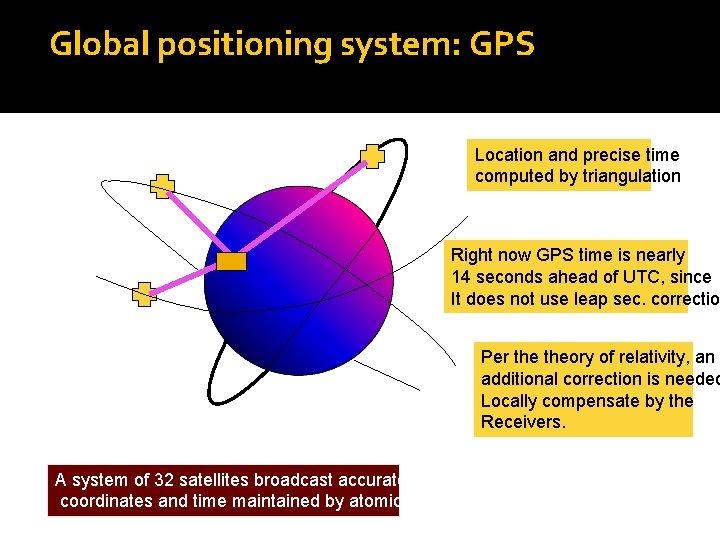Global positioning system: GPS Location and precise time computed by triangulation Right now GPS time is nearly 14 seconds ahead of UTC, since It does not use leap sec. correctio Per theory of relativity, an additional correction is needed Locally compensate by the Receivers. A system of 32 satellites broadcast accurate spatial coordinates and time maintained by atomic clocks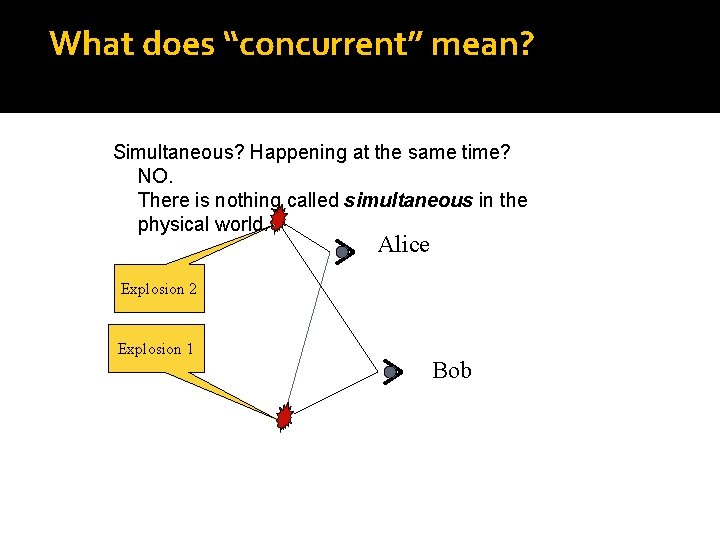What does “concurrent” mean? Simultaneous? Happening at the same time? NO. There is nothing called simultaneous in the physical world. Alice Explosion 2 Explosion 1 BobSequential and Concurrent events Sequential = Totally ordered in time. Total ordering is feasible in a single process that has only one clock. This is not true in a distributed system. Two issues are important here: ¨ How to synchronize physical clocks? ¨ Can we define sequential and concurrent events without using physical clocks?Causality helps identify sequential and concurrent events without using physical clocks. Joke Re: joke ( implies causally ordered before or happened before) Message sent message received Local ordering: a b c (based on the local clock)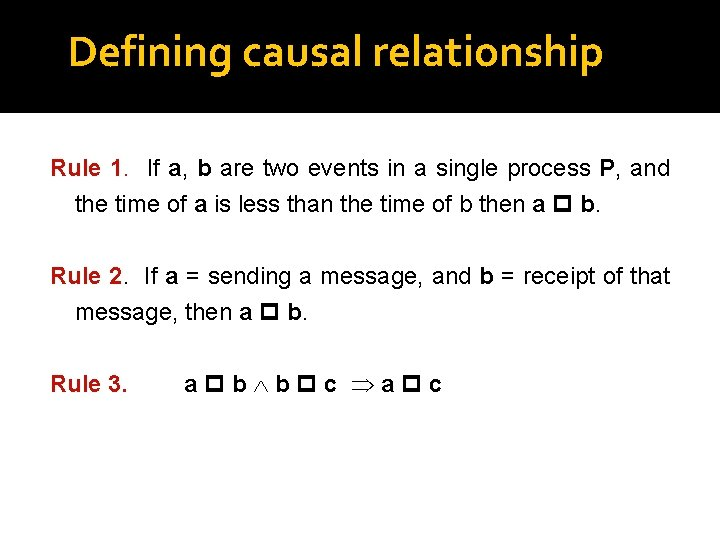Defining causal relationship Rule 1. If a, b are two events in a single process P, and the time of a is less than the time of b then a b. Rule 2. If a = sending a message, and b = receipt of that message, then a b. Rule 3. a b b c a c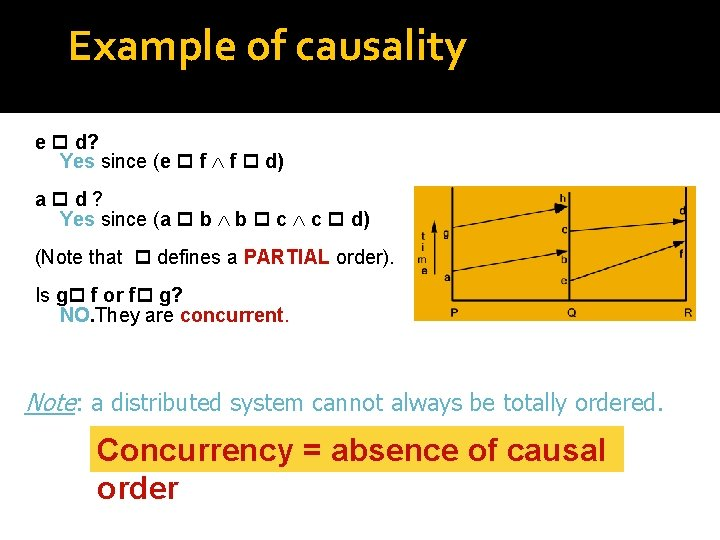Example of causality e d? Yes since (e f f d) a d? Yes since (a b b c c d) (Note that defines a PARTIAL order). Is g f or f g? NO. They are concurrent. Note: a distributed system cannot always be totally ordered. Concurrency = absence of causal order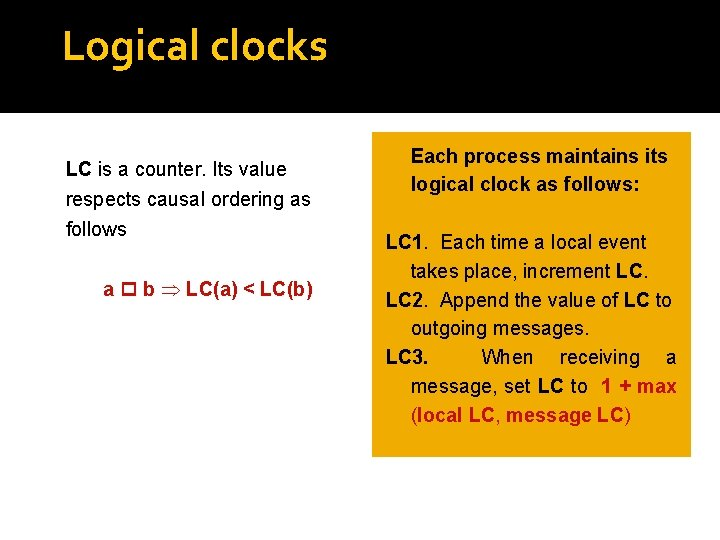Logical clocks LC is a counter. Its value respects causal ordering as follows a b LC(a) < LC(b) Each process maintains its logical clock as follows: LC 1. Each time a local event takes place, increment LC. LC 2. Append the value of LC to outgoing messages. LC 3. When receiving a message, set LC to 1 + max (local LC, message LC)Total order in a distributed system Total order is important for some applications like scheduling (firstcome first served). But total order does not exist! What can we do? Strengthen the causal order to define a total order (<<) among events. Use LC to define total order (in case two LC’s are equal, process id’s will be used to break the tie). Let a, b be events in processes i and j respectively. Then a << b iff -- LC(a) < LC(b) OR -- LC(a) = LC(b) and i < j a b a << b, but the converse is not true. The value of LC of an event is called its timestamp.Vector clock Causality detection can be an important issue in applications like group communication. Logical clocks do not detect causal ordering. Vector clocks do. Mapping VC from events to integer arrays, and an order < such that for any pair of a, b: a b VC(a) < VC(b) C may receive Re: joke before joke, which is bad!Implementing VC {Actions of process j} jth component of VC 1. Increment VC[j] for each local event. 2. Append the local VC to every outgoing message. 3. When a process j receives a message with a vector timestamp T from another process, first increment the jth component VC[j] of its own vector clock, and then update it as follows: k: 0 ≤ k ≤N-1: : VC[k] : = max (T[k], VC[k]).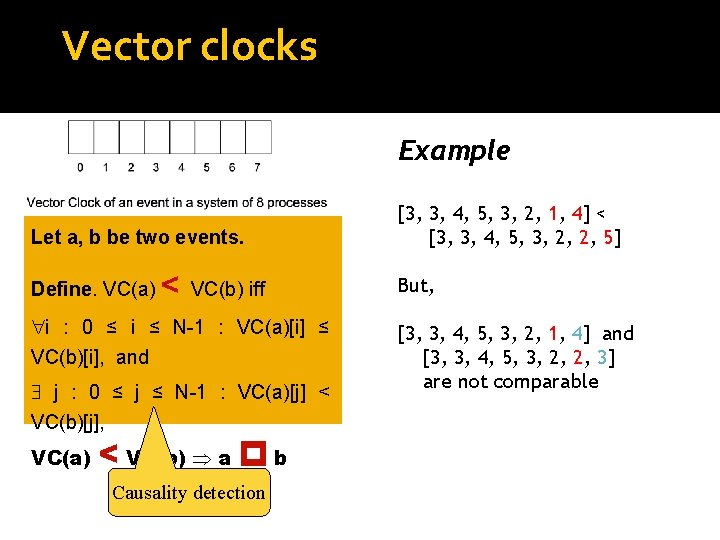Vector clocks Example Let a, b be two events. Define. VC(a) < VC(b) iff i : 0 ≤ i ≤ N-1 : VC(a)[i] ≤ VC(b)[i], and j : 0 ≤ j ≤ N-1 : VC(a)[j] < VC(b)[j], VC(a) < VC(b) a b Causality detection [3, 3, 4, 5, 3, 2, 1, 4] < [3, 3, 4, 5, 3, 2, 2, 5] But, [3, 3, 4, 5, 3, 2, 1, 4] and [3, 3, 4, 5, 3, 2, 2, 3] are not comparable